# LINQ Intersect Method in C#

## LINQ Intersect Method in C# with Examples

In this article, I will discuss the LINQ Intersect Method using C# with Examples. Please read our previous article discussing the LINQ Except Method in C# with Examples. The LINQ Intersect Method in C# is used to find the set intersection of two sequences. As part of this article, I will discuss the following pointers.

1. What is the LINQ Intersect Method?
2. Examples to Understand LINQ Intersect Method with Value Type.
3. What happens if any sequences are null while performing the Intersect Operation?
4. LINQ Intersect() Method Example with String Array using C#.
5. LINQ Intersect Method with Complex Type in C#.
6. Using IEqualityComparer Comparer with LINQ Intersect Method in C#.
7. Using Anonymous Type with Intersect Method in C#.
8. Overriding Equals() and GetHashCode() Methods.
9. Implementing IEquatble<T> Interface.
10. When to Use the LINQ Intersect Method in C#?
##### What is the LINQ Intersect Method?

The LINQ Intersect Method in C# returns the common elements from both collections. That means the elements that are present in both data sources are going to be returned by the Intersect Method. The Intersect method is part of the System.Linq namespace can be applied to any type implementing IEnumerable<T>. There are two overloaded versions available for the Intersect Method in C#. They are as follows.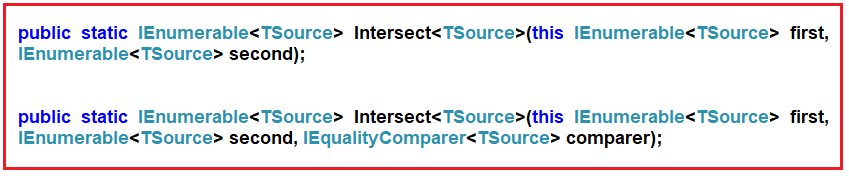The only difference between the two LINQ Intersect methods is that the second overloaded version uses IEqualityComparer as an argument. That means when working with Complex Types, we can use the overloaded method to work as expected, which takes the IEqualityComparer parameter.

##### Key Features of LINQ Intersect Method:
• Default Equality Comparer: By default, Intersect uses the default equality comparer for the type of elements in the sequences.
• Custom Equality Comparer: You can provide a custom equality comparer if you need to control how the elements are compared for equality.
• Deferred Execution: The Intersect uses deferred execution. The actual intersection computation is delayed until you iterate over the query.
• Uniqueness in Result: The result of Intersect contains no duplicate elements, even if the input sequences contain duplicates.
##### Examples to Understand LINQ Intersect Method with Value Type

Let us understand the LINQ Intersect Method with an example. Please have a look at the below image. As you can see in the image below, we have two integer data sources, i.e., DataSource 1 and Data Source 2. DataSource 1 contains elements 1, 2, 3, 4, 5, and 6, and DataSource 2 contains elements 1, 3, 5, 8, 9, and 10. If we want to retrieve the elements, such as 1, 3, and 5, which exist in both data sources, then we need to use the LINQ Intersect method.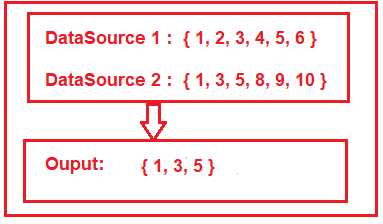##### LINQ Intersect() Method Example using Method and Query Syntax:

The following example shows the use of the LINQ Intersect() Method using both Method and Query Syntax to fetch the common elements that exist in both collections. There is no such operator called Intersect in query syntax, so we need to use mixed syntax, i.e., both the query and method syntax, to achieve the same.

```using System.Collections.Generic;
using System;
using System.Linq;
namespace LINQDemo
{
class Program
{
static void Main(string[] args)
{
List<int> dataSource1 = new List<int>() { 1, 2, 3, 4, 5, 6 };
List<int> dataSource2 = new List<int>() { 1, 3, 5, 8, 9, 10 };

//Method Syntax
var MS = dataSource1.Intersect(dataSource2).ToList();

//Query Syntax
var QS = (from num in dataSource1
select num)
.Intersect(dataSource2).ToList();

foreach (var item in MS)
{
Console.WriteLine(item);
}

}
}
}```

Run the application, and you will see the output as 1 3 5.

##### What happens if any sequences are null while performing the Intersect Operation?

The Intersect Method will throw an exception if any sequences are null. In the example below, the second sequence is null, and performing the Intersect operation using the Intersect Method will throw an exception.

```using System.Collections.Generic;
using System;
using System.Linq;
namespace LINQDemo
{
class Program
{
static void Main(string[] args)
{
List<int> dataSource1 = new List<int>() { 1, 2, 3, 4, 5, 6 };
List<int> dataSource2 = null;

//Method Syntax
var MS = dataSource1.Intersect(dataSource2).ToList();

foreach (var item in MS)
{
Console.WriteLine(item);
}

}
}
}```

Now run the application, and you will get the following exception.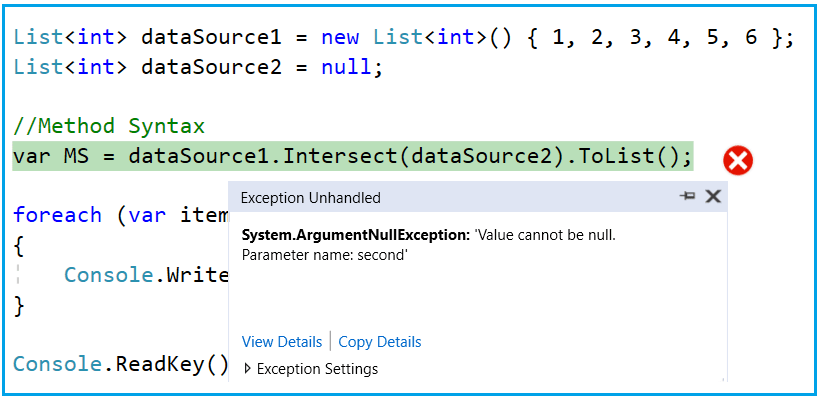##### LINQ Intersect() Method Example with String Array using C#:

Let us see the examples to understand how the Intersect Method works with string collection. In the example below, we have two arrays of countries, and our requirement is to return the common countries, i.e., the countries that exist in both collections.

```using System;
using System.Linq;
namespace LINQDemo
{
class Program
{
static void Main(string[] args)
{
string[] dataSource1 = { "India", "USA", "UK", "Canada", "Srilanka" };
string[] dataSource2 = { "India", "uk", "Canada", "France", "Japan" };

//Method Syntax
var MS = dataSource1.Intersect(dataSource2).ToList();

//Query Syntax
var QS = (from country in dataSource1
select country)
.Intersect(dataSource2).ToList();

foreach (var item in QS)
{
Console.WriteLine(item);
}

}
}
}```

Now run the application, and you will get the following output.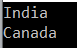As you can see, it displays only India and Canada. If you look at our collections, you can see the country “UK” is present in both collections, but the Intersect method did not fetch that country. This is because the default comparer that is being used by the Intersect method is case-insensitive.

So, if you want to ignore the case-sensitive, you need to use the other overloaded version of the Intersect() method, which takes IEqualityComparer as an argument. So, modify the program as shown below, where we pass StringComparer as an argument to the Intersect() method, which will ignore the case sensitivity while comparing the values.

```using System;
using System.Linq;
namespace LINQDemo
{
class Program
{
static void Main(string[] args)
{
string[] dataSource1 = { "India", "USA", "UK", "Canada", "Srilanka" };
string[] dataSource2 = { "India", "uk", "Canada", "France", "Japan" };

//Method Syntax
var MS = dataSource1.Intersect(dataSource2, StringComparer.OrdinalIgnoreCase).ToList();

//Query Syntax
var QS = (from country in dataSource1
select country)
.Intersect(dataSource2, StringComparer.OrdinalIgnoreCase).ToList();

foreach (var item in QS)
{
Console.WriteLine(item);
}

}
}
}```

Now run the application, and it will display the data as expected, as shown in the below image.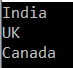##### LINQ Intersect Method with Complex Type in C#:

The LINQ Intersect() Method, like other Set Methods (such as Distinct and Expect), also works differently when working with complex data types such as Product, Employee, Student, etc. Let us understand this with an example. Create a class file named Student.cs and copy and paste the following code.

```namespace LINQDemo
{
public class Student
{
public int ID { get; set; }
public string Name { get; set; }
}
}
```

This is a very simple student class with just two properties. Let’s say we have the following two data sources.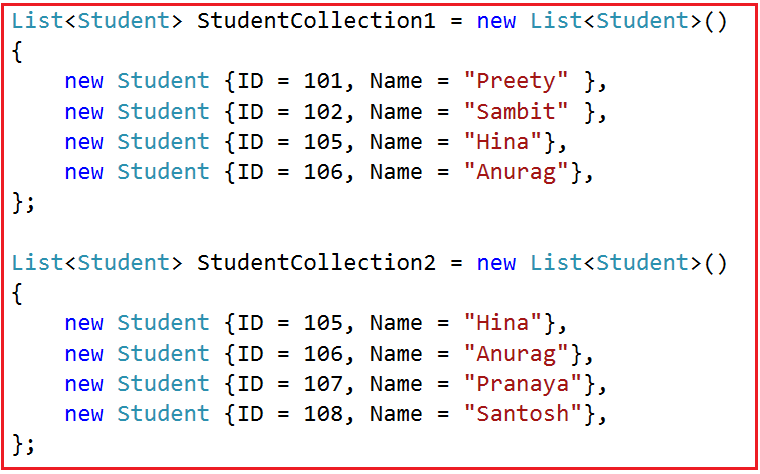As you can see in the above image, we have two student data collections. And if you notice, we have two students who appear in both collections. Our requirement is to fetch all the student names which are present in both collections. That is the common student’s names from both collections. To achieve this, modify the Main method of the Program class as follows. In the example below, from the first data source, we are fetching the student names by using the select method, then we use the Intersect method, and then we are fetching the student names from the second data source.

```using System.Collections.Generic;
using System;
using System.Linq;
namespace LINQDemo
{
class Program
{
static void Main(string[] args)
{
List<Student> StudentCollection1 = new List<Student>()
{
new Student {ID = 101, Name = "Preety" },
new Student {ID = 102, Name = "Sambit" },
new Student {ID = 105, Name = "Hina"},
new Student {ID = 106, Name = "Anurag"},
};

List<Student> StudentCollection2 = new List<Student>()
{
new Student {ID = 105, Name = "Hina"},
new Student {ID = 106, Name = "Anurag"},
new Student {ID = 107, Name = "Pranaya"},
new Student {ID = 108, Name = "Santosh"},
};

//Method Syntax
var MS = StudentCollection1.Select(x => x.Name)
. Intersect(StudentCollection2.Select(y => y.Name)).ToList();

//Query Syntax
var QS = (from std in StudentCollection1
select std.Name)
. Intersect(StudentCollection2.Select(y => y.Name)).ToList();

foreach (var name in MS)
{
Console.WriteLine(name);
}

}
}
}```

When you run the above code, you will get the output as expected, as shown in the below image: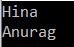##### More Complex Examples to Understand Linq Intersect Example:

Now, our requirement is to select all the students’ information in both collections. To do this, let us modify the program class as shown below.

```using System.Collections.Generic;
using System;
using System.Linq;
namespace LINQDemo
{
class Program
{
static void Main(string[] args)
{
List<Student> StudentCollection1 = new List<Student>()
{
new Student {ID = 101, Name = "Preety" },
new Student {ID = 102, Name = "Sambit" },
new Student {ID = 105, Name = "Hina"},
new Student {ID = 106, Name = "Anurag"},
};

List<Student> StudentCollection2 = new List<Student>()
{
new Student {ID = 105, Name = "Hina"},
new Student {ID = 106, Name = "Anurag"},
new Student {ID = 107, Name = "Pranaya"},
new Student {ID = 108, Name = "Santosh"},
};

//Method Syntax
var MS = StudentCollection1.Intersect(StudentCollection2).ToList();

//Query Syntax
var QS = (from std in StudentCollection1
select std).Intersect(StudentCollection2).ToList();

foreach (var student in MS)
{
Console.WriteLine(\$" ID : {student.ID} Name : {student.Name}");
}

}
}
}```

Once you run the application, then it will not display any data. This is because the default comparer, used by the LINQ Intersect Method for comparison, only checks whether two object references are equal and not the individual property values of the complex object. In this case, each student object has a different reference, so it does not return any student data. As we already discussed, we can overcome this problem in many ways. Let us understand each way one by one.

##### Using IEqualityComparer Comparer with LINQ Intersect Method in C#:

In this approach, we need to create a class, and then we need to implement the IEqualityComparer interface. We need to provide the implementation for the Equals and GetHashCode methods. So, create a class file named StudentComparer.cs and copy and paste the following code.

```using System.Collections.Generic;
namespace LINQDemo
{
public class StudentComparer : IEqualityComparer<Student>
{
public bool Equals(Student x, Student y)
{
return x.ID == y.ID && x.Name == y.Name;
}

public int GetHashCode(Student obj)
{
return obj.ID.GetHashCode() ^ obj.Name.GetHashCode();
}
}
}
```

Next, we need to create an instance of the StudentComparer class, and then we need to pass that instance to the Intersect method. So, modify the Main method of the Program class as follows.

```using System.Collections.Generic;
using System;
using System.Linq;
namespace LINQDemo
{
class Program
{
static void Main(string[] args)
{
List<Student> StudentCollection1 = new List<Student>()
{
new Student {ID = 101, Name = "Preety" },
new Student {ID = 102, Name = "Sambit" },
new Student {ID = 105, Name = "Hina"},
new Student {ID = 106, Name = "Anurag"},
};

List<Student> StudentCollection2 = new List<Student>()
{
new Student {ID = 105, Name = "Hina"},
new Student {ID = 106, Name = "Anurag"},
new Student {ID = 107, Name = "Pranaya"},
new Student {ID = 108, Name = "Santosh"},
};

StudentComparer studentComparer = new StudentComparer();

//Method Syntax
var MS = StudentCollection1
.Intersect(StudentCollection2, studentComparer).ToList();

//Query Syntax
var QS = (from std in StudentCollection1
select std)
.Intersect(StudentCollection2, studentComparer).ToList();

foreach (var student in QS)
{
Console.WriteLine(\$" ID : {student.ID} Name : {student.Name}");
}

}
}
}
```

With the above changes in place, run the application, and you will get the output as expected, as shown in the below image.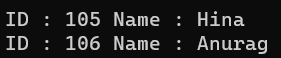##### Using Anonymous Type with Intersect Method in C#:

In this approach, we must select all the individual properties to an anonymous type using the LINQ select operator or Select Extension Method. The following program does exactly the same thing.

```using System.Collections.Generic;
using System;
using System.Linq;
namespace LINQDemo
{
class Program
{
static void Main(string[] args)
{
List<Student> StudentCollection1 = new List<Student>()
{
new Student {ID = 101, Name = "Preety" },
new Student {ID = 102, Name = "Sambit" },
new Student {ID = 105, Name = "Hina"},
new Student {ID = 106, Name = "Anurag"},
};

List<Student> StudentCollection2 = new List<Student>()
{
new Student {ID = 105, Name = "Hina"},
new Student {ID = 106, Name = "Anurag"},
new Student {ID = 107, Name = "Pranaya"},
new Student {ID = 108, Name = "Santosh"},
};

//Method Syntax
var MS = StudentCollection1.Select(x => new { x.ID, x.Name })
.Intersect(StudentCollection2.Select(x => new { x.ID, x.Name })).ToList();

//Query Syntax
var QS = (from std in StudentCollection1
select new {std.ID, std.Name })
.Intersect(StudentCollection2.Select(x => new { x.ID, x.Name })).ToList();

foreach (var student in MS)
{
Console.WriteLine(\$" ID : {student.ID} Name : {student.Name}");
}

}
}
}```

With the above changes in place, run the application code again, and you will get the output as expected, as shown in the below image.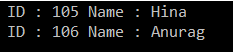##### Overriding Equals() and GetHashCode() Methods within the Student Class

This is the third approach, and in this approach, we need to override the Equals and GetHashCode() in the Student class. By default, any type (predefined or user-defined) .NET Framework is inherited from the Object class. That means the Student class is also inherited from the Object class. So, we can override the Equals() and GetHashCode() methods of the Object class within the Student class. So, modify the Student class as shown below. We are overriding the Equals() and GetHashCode() methods here.

```namespace LINQDemo
{
public class Student
{
public int ID { get; set; }
public string Name { get; set; }

public override bool Equals(object obj)
{
//As the obj parameter type is object, so we need to
//cast it to Student Type
return this.ID == ((Student)obj).ID && this.Name == ((Student)obj).Name;
}

public override int GetHashCode()
{
//Get the ID hash code value
int IDHashCode = this.ID.GetHashCode();
//Get the string HashCode Value
//Check for null refernece exception
int NameHashCode = this.Name == null ? 0 : this.Name.GetHashCode();
return IDHashCode ^ NameHashCode;
}
}
}
```

Next, we need to modify the Main method of the Program class, as shown below. Here, we need to use the overloaded version of the Intersect method, which does not take any parameter.

```using System.Collections.Generic;
using System;
using System.Linq;
namespace LINQDemo
{
class Program
{
static void Main(string[] args)
{
List<Student> StudentCollection1 = new List<Student>()
{
new Student {ID = 101, Name = "Preety" },
new Student {ID = 102, Name = "Sambit" },
new Student {ID = 105, Name = "Hina"},
new Student {ID = 106, Name = "Anurag"},
};

List<Student> StudentCollection2 = new List<Student>()
{
new Student {ID = 105, Name = "Hina"},
new Student {ID = 106, Name = "Anurag"},
new Student {ID = 107, Name = "Pranaya"},
new Student {ID = 108, Name = "Santosh"},
};

//Method Syntax
var MS = StudentCollection1.Intersect(StudentCollection2).ToList();

//Query Syntax
var QS = (from std in StudentCollection1
select std).Intersect(StudentCollection2).ToList();

foreach (var student in MS)
{
Console.WriteLine(\$" ID : {student.ID} Name : {student.Name}");
}

}
}
}
```

Now run the application, and again, you will get the same output as the previous two examples, as shown in the below image.##### Implementing IEquatble<T> Interface in Student Class

This is the last approach, and, in this approach, we need to implement the IEquatble<T> Interface in the Student Class. We need to implement the Equals Method of the IEquatble<T> Interface, and we also need to override the GetHashCode method of the Object class. So, modify the Student class as shown below.

```using System;
namespace LINQDemo
{
public class Student : IEquatable<Student>
{
public int ID { get; set; }
public string Name { get; set; }

public bool Equals(Student other)
{
return this.ID.Equals(other.ID) && this.Name.Equals(other.Name);
}
public override int GetHashCode()
{
int IDHashCode = this.ID.GetHashCode();
int NameHashCode = this.Name == null ? 0 : this.Name.GetHashCode();
return IDHashCode ^ NameHashCode;
}
}
}
```

As you can see, here we have done two things. First, we implement the Equals method of the IEquatable interface, and then we override the GetHashCode method of the Object class. With the above changes in place, now modify the Main Method of the Program class as shown below.

```using System.Collections.Generic;
using System;
using System.Linq;
namespace LINQDemo
{
class Program
{
static void Main(string[] args)
{
List<Student> StudentCollection1 = new List<Student>()
{
new Student {ID = 101, Name = "Preety" },
new Student {ID = 102, Name = "Sambit" },
new Student {ID = 105, Name = "Hina"},
new Student {ID = 106, Name = "Anurag"},
};

List<Student> StudentCollection2 = new List<Student>()
{
new Student {ID = 105, Name = "Hina"},
new Student {ID = 106, Name = "Anurag"},
new Student {ID = 107, Name = "Pranaya"},
new Student {ID = 108, Name = "Santosh"},
};

//Method Syntax
var MS = StudentCollection1.Intersect(StudentCollection2).ToList();

//Query Syntax
var QS = (from std in StudentCollection1
select std).Intersect(StudentCollection2).ToList();

foreach (var student in MS)
{
Console.WriteLine(\$" ID : {student.ID} Name : {student.Name}");
}

}
}
}
```

Now, run the application, and you will also get the same output as expected, as shown in the below image.##### When to Use the LINQ Intersect Method in C#?

The LINQ Intersect method in C# is particularly useful in scenarios where you need to find common elements between two sequences. Here are some typical use cases:

• Identifying Common Elements: The most direct use of Intersect is finding elements in both collections. This can be useful in various domains, such as finding common tags between two sets of articles, common interests between users, or shared items in inventory lists.
• Data Analysis and Comparison: Intersect can be used to compare datasets and identify overlapping data points in data analysis. For example, it can be used to find common customers between two different sales periods or shared characteristics in demographic studies.
• Filtering Data Based on Another Collection: Intersect can act as a filter to refine a collection based on the presence of its elements in another collection. This is useful in scenarios where you have a master list and want to see which elements of a subset list are present.
• Database Queries: When working with LINQ to Entities or LINQ to SQL, Intersect can translate to an efficient SQL INTERSECT query, allowing you to perform set operations at the database level.
• Testing and Validation: In testing scenarios, Intersect can be used to verify that a given set of results contains all expected elements by intersecting it with a set of expected values.
• Ensuring Uniqueness in Results: Since Intersect returns a set of distinct elements that appear in both sequences, it can be used to ensure that the resulting collection does not have duplicates.

In the next article, I will discuss the LINQ Union Method using C# with Examples. In this article, I try to explain the LINQ Intersect Method in C# with Examples, and I hope you enjoy this article.

### 1 thought on “LINQ Intersect Method in C#”

1.Awesome tutorial! Could you please add specification on which way is the best and what scenarios?